Atomic Masses

Protons, electrons and neutrons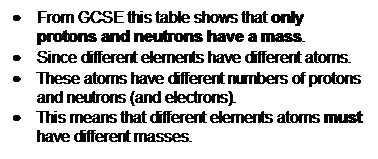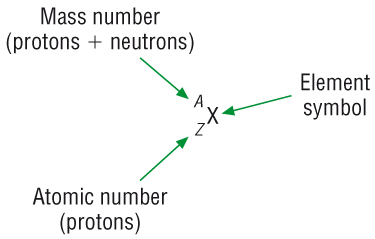·        So an atom of one element must have a different mass from another element, we call this the Mass Number.

·        The number of protons determines which element an atom is and the bottom number tell us this, we call this the Atomic number.

Examples:-

1) Lithium

 7 Li 3

The top number is the Mass number.  This means that the total number of protons and neutrons are 7.

The bottom number is the Atomic number.  This is the number of protons.

Because an atom is neutral, this means that this is also the number of

electrons.  This atom has 3 protons and 3 electrons.

If we take the Atomic number (Z) from the Mass number (A) we get the number of

neutrons.  7-3=4 neutrons.

2)  Nitrogen

 14 N 7

An atom of nitrogen is twice as heavy as an atom of Lithium.

The top number is the mass number.  This means that the total number of protons and neutrons are 14.

The bottom number is the Atomic number.  This is the number of protons.

Because an atom is neutral, this means that this is also the number of

electrons.  This atom has 7 protons and 7 electrons.

If we take the Atomic number (Z) from the Mass number (A) we get the number of

neutrons.  14-7=7 neutrons.

Isotopes

An atom of the same element that has the same number of protons and electrons but a different number of neutrons.

• As the atom has the same number of protons and electrons it will have the same chemical properties.
• They are all hydrogen atoms because they all have the same number of protons
• Hydrogen can be used as an example:-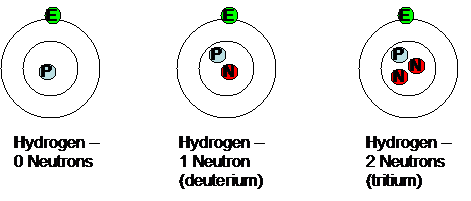Measurement of relative masses

·        Because Chemistry is about reacting ratios we have to measure the amount of reacting particles of each reactant.

·        We now know that each atom (and therefore molecules) have different masses (because they have different numbers of protons and neutrons), we have to be able to weigh out a number of particles of reactants to react with each other.

·        Since atoms are so small we give them a mass scale of their own.

·        This scale is called:

Unified atomic mass unit, u:        1u   =    1.66 x 10-24g

·        This is basically the masses of a proton / neutron.

·        The mass of a carbon - 12 atom = 12u

·        The mass of 1/12th of a carbon - 12 atom = 1u

·        We have to state the Atomic mass number of the atom as elements usually have isotopes:

there is a carbon - 13 atom containing an extra neutron, this would have an mass of 13u!!

·        This is not the only thing we have to be careful of, there are 4 different types of masses and we have to use the correct one depending what we are referring to:

1)  Relative isotopic mass:

·        We use this one when we are only referring to one isotope of an element

16O has a mass of 16u

2)  Relative atomic mass:

·        We use this one when we are referring to the mixture of naturally occurring isotopes in of an element (the average by % - later)

3)  Relative molecular mass, (Mr):

·        We use this one when we are referring to simple molecules.  Basically covalently bonded molecules, Cl2, H2O

water, H2O has a mass of 18u

H    1    x    2    =      2

O    16  x    1    =    16

18

4)  Relative formula mass:

·        We use this one when we are referring to ionic compounds and giant covalent compounds.  Such as CaBr2, SiO2

Calcium bromide, CaBr2 has a mass of 199.9u

Ca    40.1    x    1    =      40.1

Br     79.9    x    2    =    159.8

199.9u

·        However for convenience we often use relative molecular mass instead.

## Calculating Relative Atomic Mass (RAM) from % abundance)

·        Use the formula:

RAM = (%  x  Ar)  +  (%  x  Ar)  +  ....

100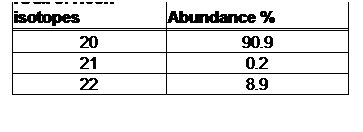·        Mass spectroscopy is the analytical method we use to obtain the % abundances for the isotopes.

Worked example:

RAM = (90.9  x  20)  +  (0.2  x  21)  +  (8.9  x  22)

100

RAM = 20.18u

Have a go for Si: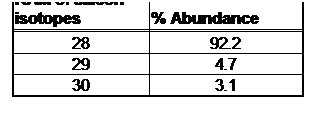Questions 1-3 p9 / 2,3 p35

Amount of substance and the mole

·        Since atoms are so small and therefore have such a small mass we have to measure them in large numbers.

·        These large numbers are called a mole.

·        The simplest way to understand the mole is to treat it as a word to describe a number:-

Dozen                       12

Ton                            100

Pony                          20

Grand                        1000

Mole                         6.02x1023  (Avogadro's constant, NA)

·        The mole is such a large number as it takes that many atoms to be able to measure a mass in g.

·        It does appear to be quite an unusual number but it has been thought out:

Basically in 12g of carbon-12 you would find 6x1023 atoms of carbon.

·        This number has been chosen to make it fit with the Atomic Masses from the Periodic Table:

1g of 1H atoms would have 6 x 1023 atoms of H

16g of 16O atoms would have 6 x 1023 atoms of O  (atom is 16 x heavier than H)

32g of 32S atoms would have 6 x 1023 atoms of S  (atom is 32 x heavier than H)

When you think about it like this it actually makes sense!!!

·        In fact if you were to measure out 6x1023 (A Mole) atoms of any element you would find that its mass is the same as its RAM:-

1 Mole of Sodium 23Na                    23g mol-1

1Mole of Magnesium 24Mg              24g mol-1

1 Mole of Iron 56Fe                           56g mol-1

·        A molecule is made up from more than 1 atom so the mass of 1 mole of that molecule will be the sum of the RAM

1 Mole of water H2O                         18g mol-1

1Mole of Sodium Chloride NaCl    58.5g mol-1

Using Moles

·        Because we can’t actually count out molecules or atoms (moles) we convert it to something we can measure i.e. mass.

·        If 1 Mole of water is 18g then 2 moles would be 36g.  3 moles would be 54g and 0.5 moles would be 9g.

·        The numbers are not always this simple so a formula helps.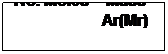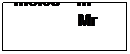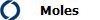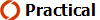Questions 1,2 p11 / 4,5 p35 / 2 p36

Types of Formula

Empirical Formula is the simplest ratio of atoms in a molecule.  Molecular formulae is the actual ratio of atoms in a molecule

·        This can be calculated using moles from percentage composition:-

Example 1

A sample of iron oxide was found to have 11.2g of iron and 4.8g of oxygen.  Calculate the formula of this compound

Fe                                           O

11.2                                        4.8

Divide by Ar                    11.2/55.8                              4.8/16

Moles                               0.2                                           0.3

Divide by smallest          0.2/0.2                                    0.3/0.2

Ratio                                1                           :                  1.5

Ratio                                2                           :                  3

Fe2O3

Example 2

A sample of hydrocarbon was found to have 1.20g of carbon and 0.25g of hydrogen.  Calculate the Empirical formula of this compound.  Then find out the molecular formula if the Mr = 58

C                                             H

1.20                                        0.25

Divide by Ar                    1.2/12                                     0.25/1

Moles                               0.1                                           0.25

Divide by smallest          0.1/0.1                                    0.25/0.1

Ratio                                1                           :                  2.5

Ratio                                2                           :                  5

Empirical formula                                C2H5

Molecular formula                               C4H10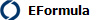Questions 1,2 p13 / 6,7 p35

Moles and gas volumes

Equal volumes of gases will have the same number of atoms / molecules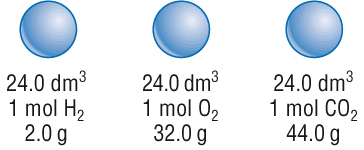·        This makes gases particularly easy to calculate 1 mole of any gas, no matter what it is, occupies the same volume (at RTP)

1 mole of any gas occupies 24dm3 (24000cm3) at room temperature and pressure.      (1dm3  =  1000cm3)

·        Again the numbers are not always simple so a general formula will help:-Questions 1-3 p15 / 8 p35 / 3 p36

Moles and solution

Concentration:

·        So far when we have looked at reacting quantities we have dealt with the mole.

·        Many reactions in chemistry involve solutions.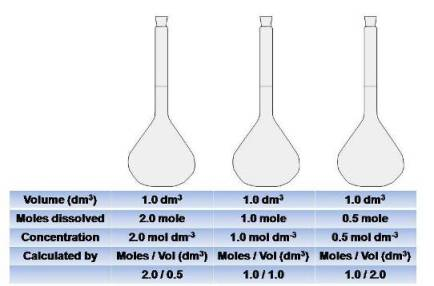·        A solution is expressed as a number of moles in 1dm3  (1 litre or 1000cm3) of solvent (usually water). ·        Concentration is the number of moles of specified entities in 1 dm3 of solution

·        We use square brackets to denote concentration [X].  (also known as molarity, M).

·        For example – a solution of sodium hydroxide has a concentration of 1.0 Mol dm-3  (1.0M)

[NaOH(aq)]  =  1.0M                This means there is 1 mole of sodium hydroxide dissolved in 1dm3 of water.

[NaCl(aq)]    =  2.0M                This means there is 2 moles of sodium chloride dissolved in 1dm3 of water.

[KOH(aq)]     =  0.5M                This means there is 0.5 moles of potassium hydroxide dissolved in 1dm3 of water.

·        This is fine so long as we keep making up 1dm3 solutions.

·        Most lab experiments only use a 100cm3 or so and making up 1000cm3 would be waste so we need to scale down: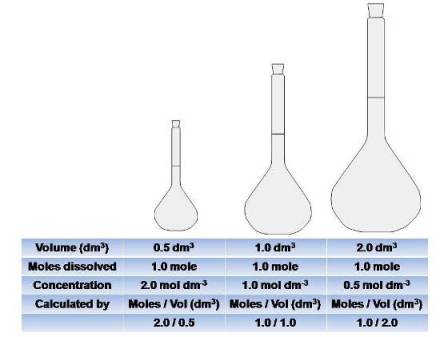·        For example:         500cm3 [NaOH(aq)]  =  1.0M                  0.5 moles of sodium hydroxide dissolved in 0.5 dm3           2000cm3 [NaCl(aq)]  =  2.0M                  4 moles of sodium chloride dissolved in 2 dm3 of water.          100cm3 [KOH(aq)]       =  0.5M                  0.05 moles of sodium hydroxide dissolved in 0.1dm3

·        The values are not usually as nice as this so we can use the following formula:-

Concentration  =  No. of moles                                    C  =  Moles

(mol dm-3)               Volume(dm3)                                           V (dm3)

·         Basically the number of moles per volume of solution.

·         We usually like our formula to have n, number of moles at the start:

No. of moles  =  Concentration (Mol dm-3)  x  Volume (dm3)                 Moles  =  C  x  V (dm3)

·         However this formula assumes we are working in dm3 and we usually work in cm3.

·         dm3 and cm3 are related by a factor of 1000 - you must convert into dm3 by dividing cm3 by 1000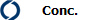Standard solutions

·        We can make solutions of known concentration using volumetric flasks.  The easiest way of learning this is to try an example.

·        We need 250cm3 of 0.1 mol dm3 solution of sodium hydroxide.

·        Use the formula to calculate the No. of moles of sodium hydroxide –

No. of moles  =  Concentration (Mol dm3)  x  Volume (dm3)                          [250 / 1000  =  0.25]

No. of moles  =  0.1 Mol dm3  x  0.25 dm3

No. of moles  =  0.025 Mol

·        Now we know how many moles of sodium carbonate we need in 250 cm3 to make a 0.800 Molar solution.

·        We now need to convert moles into a mass –

Mass  =  No. Moles x Mr                                 Mr (NaOH) =       1x23 =  23

1x16 =   16

Mass  =  0.025 x 40                                                                     1x  1 =     1

40 gMol-1

Mass  =  1g

·        Weigh this out in a beaker.

·        Dissolve in distilled water and pour into the graduated flask.

·        Add more distilled water to the beaker to wash the solute into the graduated flask.

·        Repeat the last step several times to ensure all the solute is in the graduated flask.

·        Fill the graduated flask with distilled water so the meniscus sits on the line.

·        Stopper the flask and invert several times to ensure mixing.

Mass Concentrations:

·        This is the mass in g dissolved in 1 dm3 of solution

·        This means that the concentrations are measured in g dm-3 instead

·        The concentration for the solution made above would be 1 g dm-3 as a mass concentration.

Concentrated vs diluted:

·        This is down to the amount of solute dissolved in solution:

·        Concentrated :  Lots of solute per dm3

·        Diluted :  A small amount of solute per dm3A16P:  Part 1:  Making a standard solution of ethanedoic acid

Questions 1-3 p17 / 9,10 p35 / 4 p36

Moles and reactions

·        Mole calculations can now be used to calculate reacting amounts / product amounts.

·        This is done by using the balanced chemical equation and moles calculations using masses, gas volumes and concentrations.

·        ALL of these require the use of the mole:

Mass / mole calculations:

·        Example:  2.4g Magnesium reacts in air to form magnesium oxide.  Calculate the mass of magnesium oxide made:

STEP1:  Write a balanced chemical equation and add the amounts given and question mark what you are asked to work out: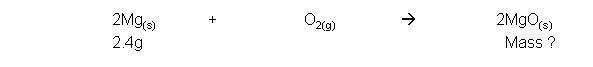Check the state symbol of your starting mass to decide which moles equation you will use - (s) - means you use Moles = mass / Ar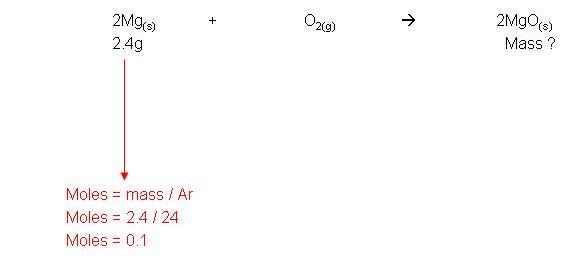Use the reacting ratios to work out how many moles you have made (or need):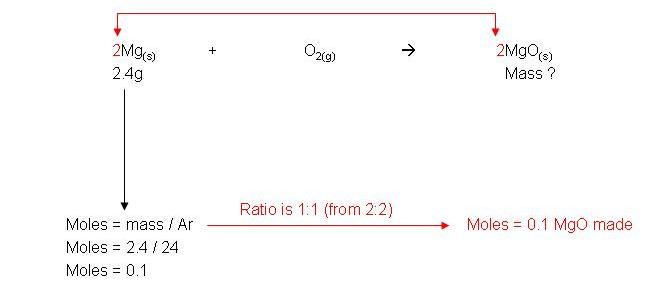Check the question/ state symbol to decide whether to convert it to mass / concentration / volume - (s) = mass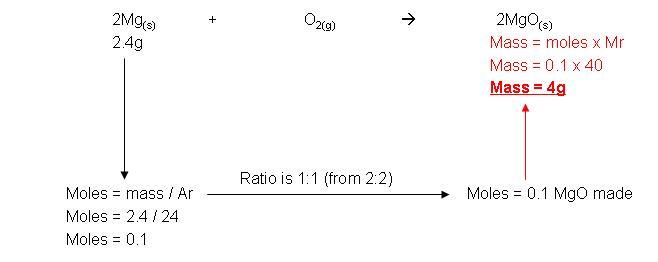Gas / mole calculations:

·        Example:  2.3g sodium reacts with excess sulphuric acid to form sodium sulphate and hydrogen gas.  Calculate the volume of hydrogen made:

STEP1:  Write a balanced chemical equation and add the amounts given and question mark what you are asked to work out: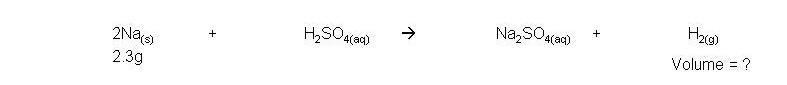Check the state symbol of your starting mass to decide which moles equation you will use - (s) - means you use Moles = mass / Ar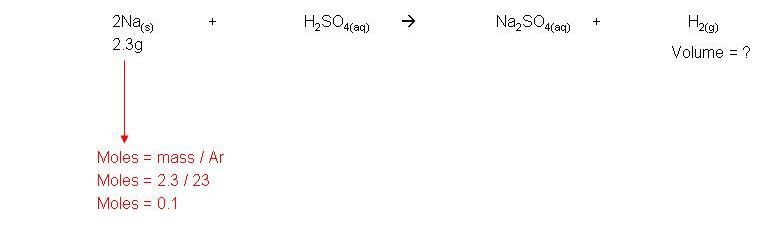Use the reacting ratios to work out how many moles you have made (or need):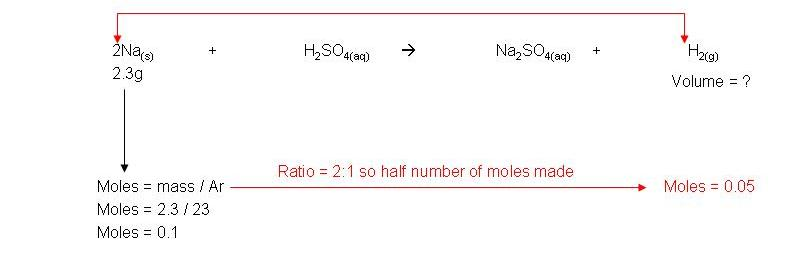Check the question/ state symbol to decide whether to convert it to mass / concentration / volume - (g) = volume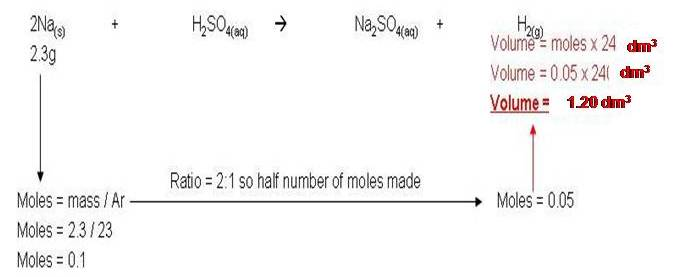Concentration / mole calculations:

·        Example:  2.3g sodium reacts with 250cm3 sulphuric acid to form sodium sulphate and hydrogen gas.  Calculate the concentration of sodium sulphate solution made:

STEP1:  Write a balanced chemical equation and add the amounts given and question mark what you are asked to work out: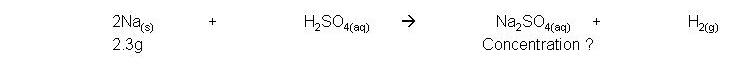Check the state symbol of your starting mass to decide which moles equation you will use - (s) - means you use Moles = mass / Ar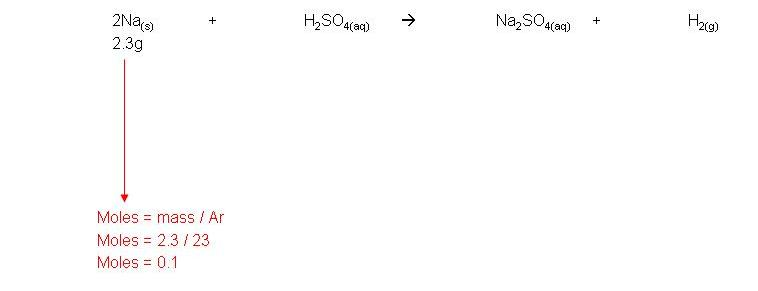Use the reacting ratios to work out how many moles you have made (or need):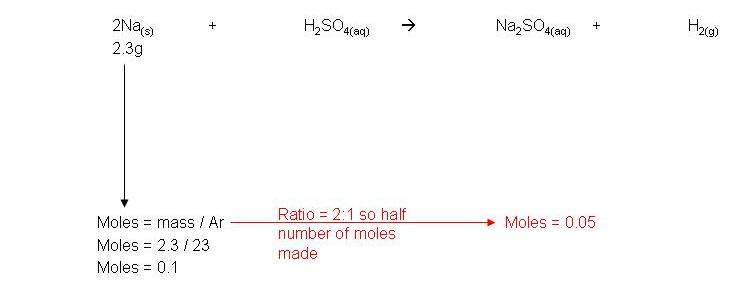Check the question/ state symbol to decide whether to convert it to mass / concentration / volume - (aq) = concentration or volume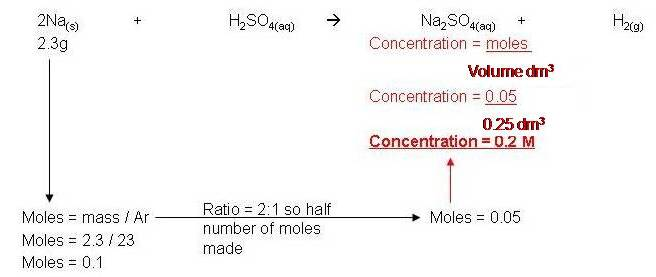A16PPart 2:  Titrating your standard solution of ethanedoic acid

Questions 1-3 p21 / 4 p36

Water of crystallisation

• Carry out the practical below and complete the 'analysis of results'

Use the fill in sheet

Interpretation:

• Coloured crystals such as blue copper sulphate contain that colour due to water locked in the crystalline structure.
• If the water is driven off then the crystals appear white.
• This water locked in the crystal is called the water of crystallisation.

Hydrated - Crystals that contain water

Anhydrous - Crystals that do not contain water

• The water can be evaporate by heat.  Some compounds will decompose so a moderate heat must be used: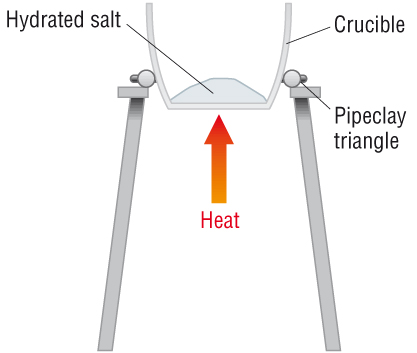• The waters in the crystal obviously have a mass and will affect the Mr of the crystal.
• The water must be written in the formula.  This is done by following a dot after the crystal formula:

CuSO4.H2O

• However most crystals will contain more than 1 mole of water per mole of crystal.
• For copper sulphate, 1 mole of copper sulphate crystals will contain 5 moles of water:

CuSO4.5H2O

• The number of moles of water per mole of crystal depends upon that crystal:

CuSO4.5H2O

CoCl2.6H2O

Na2SO4.10H2O

• The water of crystallisation can be calculated by empirical formula and by moles calculations:

From empirical formula

• Count the number of hydrogen's and divide by 2.  Do not use oxygen as this may be in the crystal formula

MgCl2H10O5    à MgCl2.5H2O

Na2CH20O13    à Na2CO3.10H2O

CaN2H8O10    à  Ca(NO3)2.4H2O

From mole calculations (example)

• From the experiment done earlier it is possible to calculate the masses of crystal and water.

Mass of hydrated MgSO4.xH2O    =    4.312g

Mass of anhydrous MgSO4    =    2.107g

• From this we can calculate the masses of the crystal and water:
• Convert these to moles of crystal and water.
• Divide through by the smallest number of moles to get a whole number ratio:
 Crystal, MgSO4 Water, H2O Masses of each 2.107g (4.312 - 2.107) 2.107g 2.205g Moles of each 2.107 / Mr 2.205 / Mr 2.107 / 120.4 2.205 / 18 0.0175 0.1225 Divide by the smallest 0.0175 / 0.0175 0.1225 / 0.0175 1 7

So the formula of hydrated MgSO4.xH2O    =    MgSO4.7H2O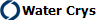Now calculate the Formula from the practical you completed earlier.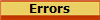Questions 1-2 p27 / 15 p35

Titrations - Volumetric analysis

·        This technique can be used to find:

Concentration

Mr

Formula

Water of crystalisation

·        To do this you react a certain volume of a solution with an unknown concentration with a solution of known concentration.

·        Using moles and reacting ratios, you can calculate the concentration of this solution.  This is known as titration.

·        The only requirement is that you can tell when one solution has completely reacted with the other.

·        Between acids and alkalis, we use indicators to let us know when the resulting solution is neutral.

·        An indicator will change colour at the ‘end point’ (neutral).

·        Common indicators are:

 Indicator Acidic colour Base colour End point colour Methyl orange Red Yellow Orange Bromothymol Blue Yellow Blue Green Phenylphthalein colourless Pink Pale pink

## Technique/procedure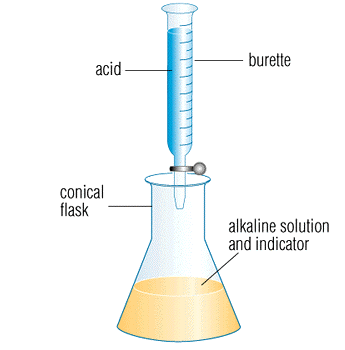·        Rinse the burette with distilled water then acid. ·        Fill the burette to the graduation mark ensuring the air bubble is removed from the tap. ·        Rinse a pipette with alkali, fill and transfer a known volume to a clean, dry conical flask. ·        Add some indicator. ·        Run the acid into the alkali and stop when the colour changes.  This is your ‘range finder’. ·        Wash the conical flask with pure water. ·        Repeat the previous steps but stop a few cm3 before the colour change. ·        Add drop wise until the first drop changes the colour of the indicator. ·        Repeat until you get 2 results that agree within 0.1cm3.

·        Record results in a table like the one below:

 Range finder / titration / cm3 Run 1 / cm3 Run 2 / cm3 2nd burette reading 1st burette reading Volume added

# Calculation – example

25cm3 of 0.01M sulphuric acid was added to exactly neutralize 50cm3 of sodium hydroxide.  Calculate the concentration of the sulphuric acid:

## 1       Write a balanced equation.

2NaOH(aq)              +             H2SO4(aq)            à        Na2SO4(aq)             +                 2H20(l)

## 2       Calculate the number of moles of acid added from the burette

No. of moles  =  Concentration (Mol dm3)  x  Volume (dm3)

(H2SO4)

No. of moles  =  0.01 Mol dm3  x  0.25 dm3

(H2SO4)

No. of moles  =  25 x 10-3 Moles

(H2SO4)

3       Use the ratio to work out the number of moles in the sample of alkali

2 : 1        NaOH : H2SO4

2x the number of moles for H2SO4

No. of moles  =   25 x 10-3 x 2

(NaOH)

No. of moles  =  50 x 10-3  Mol

(NaOH)

4           Calculate the concentration.  (Convert to g dm3 if necessary)

Concentration (Mol dm3)  =  No Moles

Volume (dm3)

Concentration (Mol dm3)  =  50 x 10-3

0.050 (dm3)

Concentration (Mol dm3)  =  1 Mol dm-3

5       Convert to g dm3 if required (usually for solubility)

Mass  =  No. moles x Mr (NaOH)

Mass  =  1 x 40

Concentration  =  40 g dm-3U1 A20:  Finding the Mr of a monobasic acidFinding the concentration of NaOHFinding the concentration of limewaterFinding the purity of a sample of sodium carbonate solutionFinding the Mr of washing soda

Questions 1-2 p29 / 17 p35

Questions 1-2 p33 / Remaining questions p35 - 37